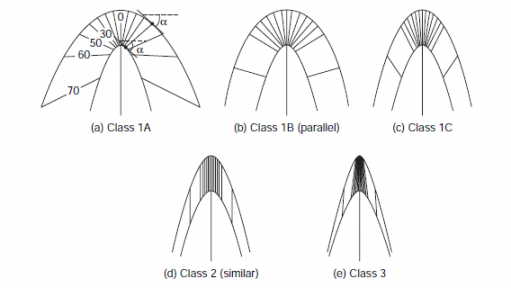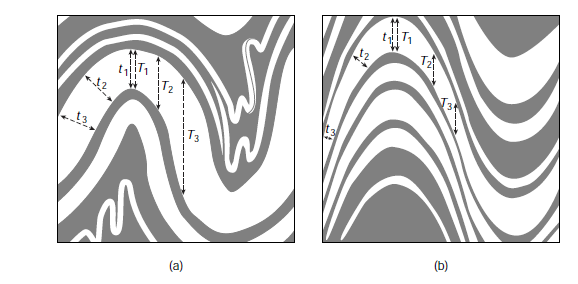## Pic of the day #31 (fold classification based on thickness)

#### Pic src: Earth structure – An introduction to structural geology and tectonicsParallel folds (a) maintain a constant layer thickness across the folded surface, meaning, t1 = t2 = t3, but the layer thickness parallel to the axial surface varies (T1 < T2 < T3). Note that parallelism must eventually break down in the cores of folds because of space limitation, which is illustrated by the small disharmonic folds in (a). In similar folds (b), the layer thickness parallel to the axial surface remains constant, so, T1 = T2 = T3, but the thickness across the folded surface varies (t1 > t2 > t3). Similar folds do not produce the space problem inherent in parallel folds.Fold classification based on dip isogon analysis. In Class 1A (a) the construction of a single dip isogon is shown, which connects the tangents to the upper and lower boundary of the folded layer with equal angle (?) relative to a reference frame; dip isogons at 10° intervals are shown for each class. Class 1 folds (a–c) have convergent dip isogon patterns; dip isogons in Class 2 folds (d) are parallel; Class 3 folds (e) have divergent dip isogon patterns. In this classification, parallel (b) and similar (d) folds are labeled as Class 1B and Class 2, respectively.

This site uses Akismet to reduce spam. Learn how your comment data is processed.